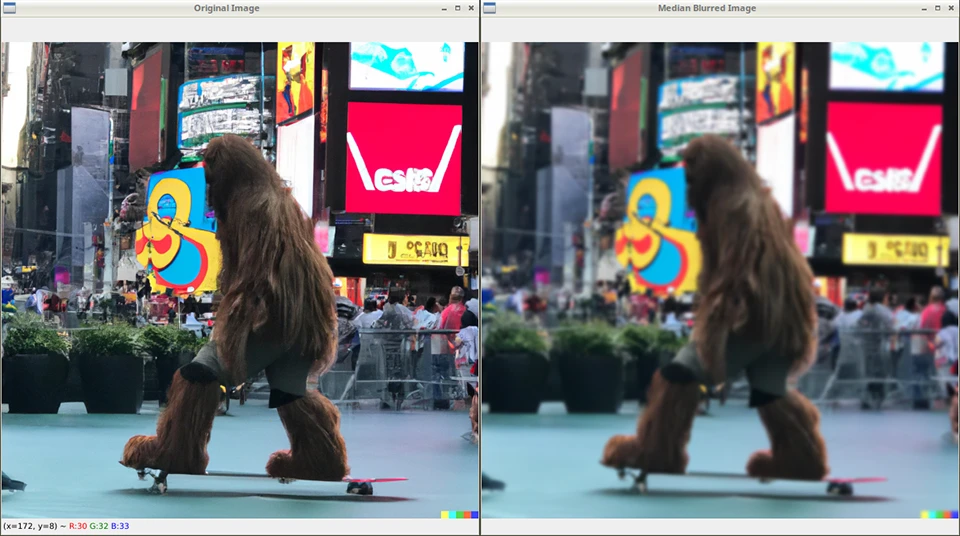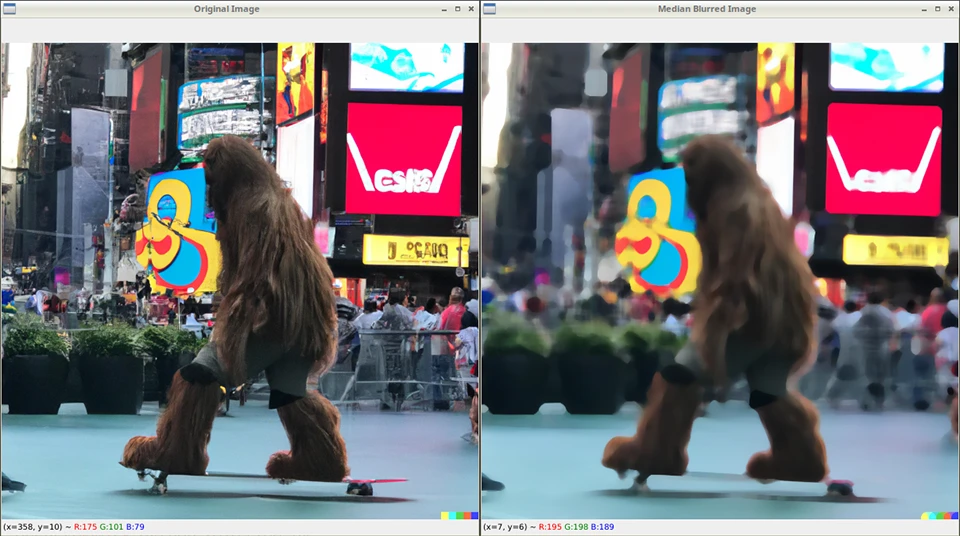Last Update: Apr 4, 2023

Updated for OpenCV 4 and Python 3, this book covers the latest on depth cameras, 3D tracking, augmented reality, and deep neural networks, helping you solve real-world computer vision problems with practical code.

##### Check out Learning OpenCV 4 Computer Vision with Python 3 now!

If you’re reading this, you’re probably curious about computer vision, or just starting out. You’ve come to the right place. In this tutorial, we’ll learn to blur images using OpenCV and Python. This is one of many OpenCV tutorials I have here.

## What is Blurring?

First off, let’s understand what blurring is. Blurring is a process where we reduce the sharpness of an image by blending the colors of neighboring pixels. Basically, we smooth out the image, which can be useful for reducing noise, improving the overall look, or creating artistic effects.

## How Does Blurring Work?

To blur an image, we need to apply a filter, which is simply a small matrix of numbers called a kernel. Don’t worry if you don’t know what a matrix is. Think of it as a grid of numbers. The kernel moves across the image, and for each pixel, it calculates the weighted average of the neighboring pixels’ colors using the kernel values.

There are different types of kernels, and today we’ll explore two common ones: the Gaussian blur and the Median blur.

## Let’s Write Some Code!

Before we start coding, make sure you have Python installed and OpenCV set up. I prefer to use Python virtual environments for my projects, and it’s easy to setup.

``````python -m venv opencvdemo
``````

Here I am naming my environment `opencvdemo` but you can name it whatever you like.

Then, activate OpenCV:

In Linux or Mac:

``````source opencvdemo/bin/activate
``````

In Windows:

``````.\opencvdemo\Scripts\activate
``````

You’ll need to install OpenCV. You can do that by using pip:

``````pip install opencv-python
``````

Now, let’s create a Python file and start by importing the necessary libraries.

I named my script `blurring.py`.

``````import cv2
import numpy as np
``````

We’ll begin by loading an image from your computer. Replace ‘sampleimage.png’ with the actual path to your image. (It can also be .jpg if you like).

``````image = cv2.imread('sampleimage.png')
``````

Here’s the sample I’m using, feel free to use it yourself. Download it hereI generated it with Dall-E, so I don’t own it.

## Gaussian Blur

Let’s start with the Gaussian blur. This kernel uses the Gaussian function, a bell-shaped curve that spreads the blur evenly around the pixel, making it look more natural.

To apply the Gaussian blur, we’ll use OpenCV’s `GaussianBlur()` function, which takes three arguments:

• The input image.
• The kernel size (width and height). They must be odd numbers.
• The standard deviation (0 means it will be calculated automatically).
``````gaussian_blur_image = cv2.GaussianBlur(image, (11, 11), 0)
``````

Now, let’s display the original image and the blurred one side by side:

``````cv2.imshow('Original Image', image)
cv2.imshow('Gaussian Blurred Image', gaussian_blur_image)
cv2.waitKey(0)
cv2.destroyAllWindows()
``````

And it looks like this:Awesome, right? Just a few lines of code and you can blur images.

## Median Blur

Now let’s do a Median blur. This kernel takes the median value (the middle value in a sorted list) of the neighboring pixels’ colors, which reduces noise while preserving edges.

We’ll use OpenCV’s `medianBlur()` function to apply the Median blur, which takes two arguments: the input image and the kernel size (an odd number).

``````median_blur_image = cv2.medianBlur(image, 11)
``````

And once again, let’s display the original and blurred images:

``````cv2.imshow('Original Image', image)
cv2.imshow('Median Blurred Image', median_blur_image)
cv2.waitKey(0)
cv2.destroyAllWindows()
``````

Let’s run it!This is a different kind of blur you may want to use.

It’s pretty easy stuff.

We know how to display these images on the screen, but that’s only part of it. What if you want to save them? It’s easy with this one line of code:

``````cv2.imwrite("output.png", median_blur_image)
``````

Of course you can change `output.png` to whatever you want.

We used the cv2.imread() function to read the image, then performed some modifications to it, and use cv.imwrite() to write it to a new image. Chances are you’ll want to use this later.

## Summary

In this tutorial, we learned about

• Blurring - what it is and how it works
• Gaussian blurs
• Median blurs
• Saving image after modification

Go ahead and experiment with this code. Change the kernel sizes and standard deviations to see how they affect blurring.

Remember, practice makes perfect.

You’ve unlocked a powerful skill in computer vision, and this is just the beginning! Often times you’ll want to apply a blur or other modifications before performing operations on an image, so now you know how.

There’s much more to explore, like edge detection, object recognition, and even facial recognition. I’ll be covering all these in future tutorials. The possibilities are endless.

Happy coding, and see you in the next tutorial.

- Jeremy

I have many OpenCV Demos on GitHub you can download and run. I build them on my Computer Vision live stream